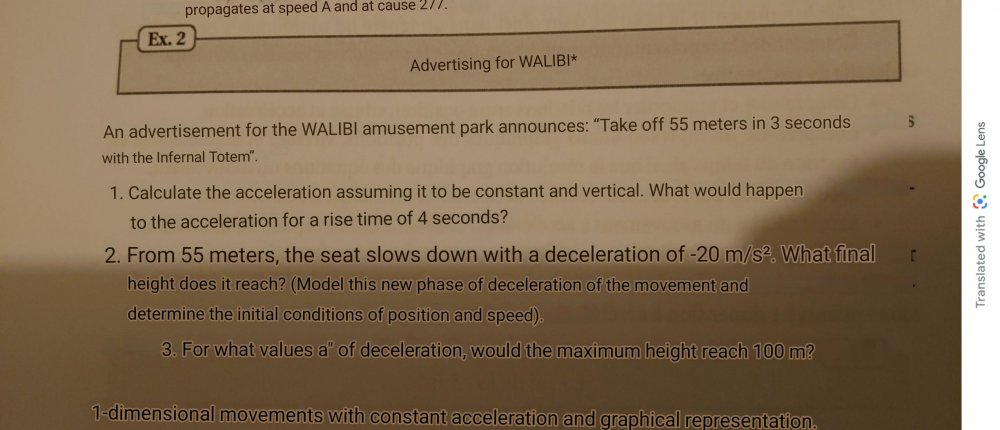# DODOma

Members

4

## Posts posted by DODOma

### Calculate acceleration given only distance and time

33 minutes ago, swansont said:

Can you post the entire question, verbatim, please?

The question is not in English so i used google translate to translate the question### Calculate acceleration given only distance and time

43 minutes ago, studiot said:

Well you haven't post the original question verbatim.

It says the rollercoaster climbs 55 meters in 3 seconds. The question is: calculate the acceleration assuming it's constant and vertical. What would happen to the acceleration if the if the time taken to climb was 4 seconds. That's the 1st question.

Just now, mistermack said:

Am I missing something? Going up or down, the acceleration would still be +g in the downward direction.

Or minus g in the upwards direction. Unless you factor in drag and friction.

It's going up.

### Calculate acceleration given only distance and time

It says It's going up vertically.

19 minutes ago, Bufofrog said:

Since roller coasters are not powered and the question says the coaster is falling vertically the acceleration should be easy to determine, think about it.

It says it's going up not coming down. In that case it would be easier a=g.

48 minutes ago, studiot said:

Since this is homework what have you done towards It ?

Have you read the question properly because as you have stated it a solution is not possible.

You say neither initial nor final velocities are supplied  so what equation were you thinking of using ?

I used this equation but there are 2 unknown values couldn't proceed, x= at^2/2 + vt + x

simplifies to a=(110 - 6v)/ 9. v is initial velocity. Because initial velocity is not given anywhere in the question, i got stuck here. So i thought i should ask a question on this forum because i never knew you could find acceleration with just distance and time given. 😅

### Calculate acceleration given only distance and time

HI, I have a question where a roller-coaster does 55m in 3s and we are asked to calculate its acceleration assuming it's vertical and constant. Other than that, there is no other information given, no initial velocity, no final velocity. So can you find the acceleration of an object with only distance and time given?

×